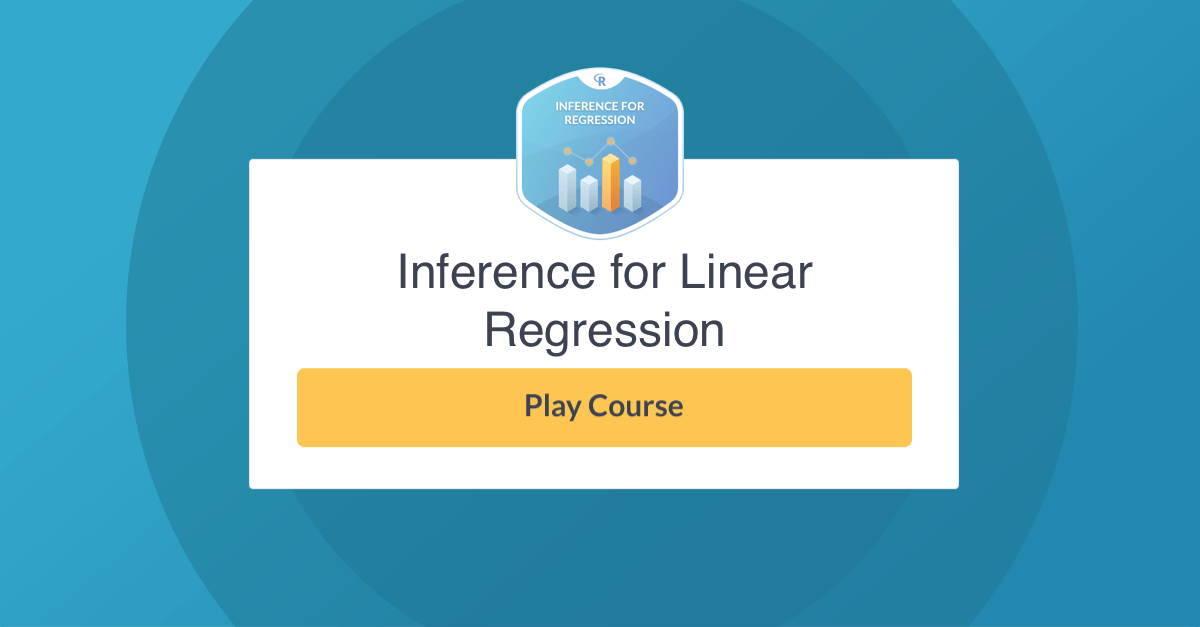# New R Course: Inference for Linear Regression

December 20, 2017
By

(This article was first published on DataCamp Community - r programming, and kindly contributed to R-bloggers)

Hello there! We’re launching a new R course today: Inference for Linear Regression by Jo Hardin!

Previously, you learned the fundamentals of both statistical inference and linear models; now, the next step is to put them together. This course gives you a chance to think about how different samples can produce different linear models, where your goal is to understand the underlying population model. From the estimated linear model, you will learn how to create interval estimates for the effect size as well as how to determine if the effect is significant. Prediction intervals for the response variable will be contrasted with estimates of the average response. Throughout the course, you’ll gain more practice with the `dplyr` and `ggplot2` packages, and you will learn about the broom package for tidying models; all three packages are invaluable in data science.

Take me to chapter 1!

Inference for Linear Regression features interactive exercises that combine high-quality video, in-browser coding, and gamification for an engaging learning experience that will make you an expert in statistical inference!What you’ll learn

1. Inferential ideas

In the first chapter, you will understand how and why to perform inferential (instead of descriptive only) analysis on a regression model.

2. Simulation-based inference for the slope parameter

In this chapter you will learn about the ideas of the sampling distribution using simulation methods for regression models.

3. t-Based Inference For the Slope Parameter

In this chapter you will learn about how to use the t-distribution to perform inference in linear regression models. You will also learn about how to create prediction intervals for the response variable.

4. Technical Conditions in linear regression

Additionally, you will consider the technical conditions that are important when using linear models to make claims about a larger population.

5. Building on Inference in Simple Linear Regression

This chapter covers topics that build on the basic ideas of inference in linear models, including multicollinearity and inference for multiple regression models.

Master statistical inference with our newest course Inference for Linear Regression!

R-bloggers.com offers daily e-mail updates about R news and tutorials on topics such as: Data science, Big Data, R jobs, visualization (ggplot2, Boxplots, maps, animation), programming (RStudio, Sweave, LaTeX, SQL, Eclipse, git, hadoop, Web Scraping) statistics (regression, PCA, time series, trading) and more...